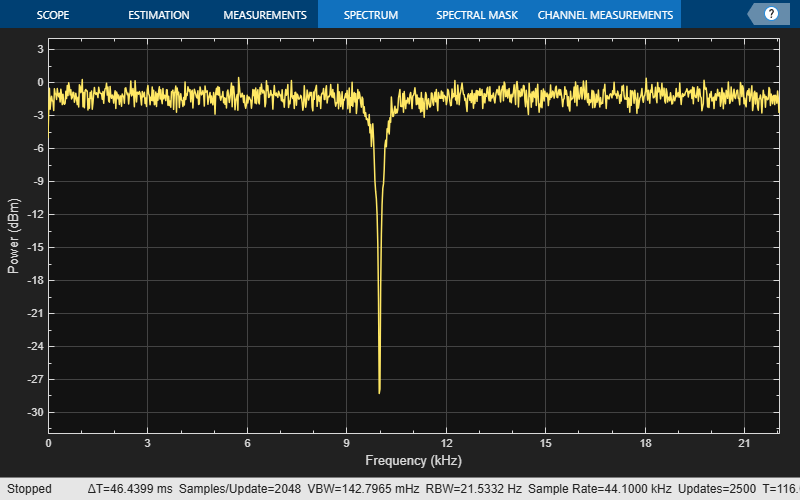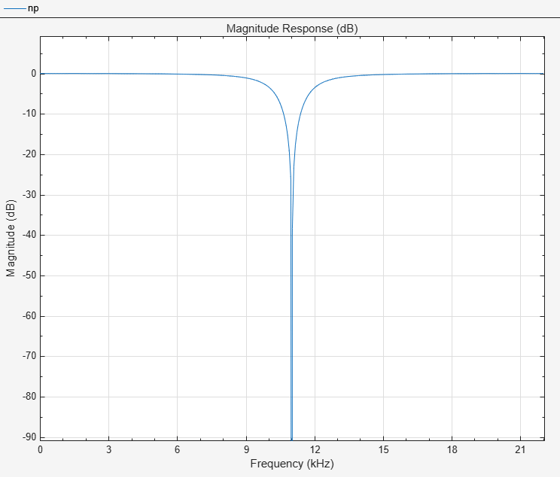dsp.NotchPeakFilter

Second-order tunable notching and peaking IIR filter

Description

The NotchPeakFilter object filters each channel of the input using IIR filter implementation.

To filter each channel of the input:

1. Create the dsp.NotchPeakFilter object and set its properties.

2. Call the object with arguments, as if it were a function.

Creation

Description

npFilter = dsp.NotchPeakFilter returns a second-order notching and peaking IIR filter that independently filters each channel of the input over time, using a specified center frequency and 3 dB bandwidth.

npFilter = dsp.NotchPeakFilter('Specification','Quality factor and center frequency') specifies the quality factor (Q factor) of the notch or peak filter instead of the 3 dB bandwidth.

npFilter = dsp.NotchPeakFilter('Specification','Coefficients') specifies the coefficient values that affect bandwidth and center frequency directly, rather than specifying the design parameters in Hz. This removes the trigonometry calculations involved when the properties are tuned.

example

npFilter = dsp.NotchPeakFilter(Name,Value) returns a notch filter with each specified property name set to the specified value. Unspecified properties have default values.

Properties

expand all

Unless otherwise indicated, properties are nontunable, which means you cannot change their values after calling the object. Objects lock when you call them, and the release function unlocks them.

If a property is tunable, you can change its value at any time.

Set the specification as 'Bandwidth and center frequency', 'Quality factor and center frequency', or 'Coefficients'.

Specify the filter’s 3 dB bandwidth as a finite positive numeric scalar in Hz. The value must be a scalar between 0 and half the sample rate.

Tunable: Yes

Dependencies

This property is applicable only if Specification is 'Bandwidth and center frequency'.

Data Types: single | double

Specify the filter’s center frequency (for both the notch and the peak) as a finite positive numeric scalar in Hz. The value must be a scalar between 0 and half the sample rate.

Tunable: Yes

Dependencies

This property is applicable only if Specification is set to 'Bandwidth and center frequency' or 'Quality factor and center frequency'.

Data Types: single | double

Specify the quality factor (Q factor) for both the notch and the peak filters. The Q factor is defined as the center frequency divided by the bandwidth. A higher Q factor corresponds to a narrower notch or peak. The Q factor should be a scalar value greater than 0.

Tunable: Yes

Dependencies

This property is applicable only if Specification is set to 'Quality factor and center frequency'.

Data Types: single | double | int8 | int16 | int32 | int64 | uint8 | uint16 | uint32 | uint64 | fi

Specify the sample rate of the input in Hz as a finite numeric scalar.

Data Types: single | double

Specify the value that determines the filter’s 3 dB bandwidth as a finite numeric scalar in the range [-1 1]. The value -1 corresponds to the maximum 3 dB bandwidth (SampleRate/4), and 1 corresponds to the minimum 3 dB bandwidth (0 Hz, an allpass filter).

Tunable: Yes

Dependencies

This property is only applicable if Specification is set to 'Coefficients'.

Data Types: single | double

Specify the coefficient that determines the filter’s center frequency as a finite numeric scalar in the range [-1 1]. The value -1 corresponds to the minimum center frequency (0 Hz), and 1 corresponds to the maximum center frequency (SampleRate/2 Hz). The default is 0, which corresponds to SampleRate/4 Hz.

Tunable: Yes

Dependencies

This property is only applicable if Specification is set to 'Coefficients'.

Data Types: single | double

Usage

Description

Y = npFilter(x) filters each channel (column) of the input signal, x, to produce the notch filter output, Y.

[Yn,Yp] = npFilter(x) filters each channel of the input signal, x, to produce the notch filter output, Yn, and peak filter output, Yp.

Input Arguments

expand all

Input signal, specified as a vector or a matrix.

Data Types: single | double
Complex Number Support: Yes

Output Arguments

expand all

Notch filter output, returned as a vector or a matrix.

Data Types: single | double
Complex Number Support: Yes

Peak filter output, returned as a vector or a matrix.

Data Types: single | double
Complex Number Support: Yes

Object Functions

To use an object function, specify the System object™ as the first input argument. For example, to release system resources of a System object named obj, use this syntax:

release(obj)

expand all

 getBandwidth Get 3 dB bandwidth getCenterFrequency Get center frequency getOctaveBandwidth Bandwidth in number of octaves getQualityFactor Get quality factor tf Transfer function
 step Run System object algorithm release Release resources and allow changes to System object property values and input characteristics reset Reset internal states of System object

Examples

collapse all

This example shows how to use dsp.NotchPeakFilter as a notch filter with center frequency of 5000 Hz and a 3 dB bandwidth of 500 Hz.

Note: This example runs only in R2016b or later. If you are using an earlier release, replace each call to the function with the equivalent step syntax. For example, myObject(x) becomes step(myObject,x).

npFilter = dsp.NotchPeakFilter('CenterFrequency',5000,'Bandwidth',500);
sa =  dsp.SpectrumAnalyzer('SampleRate',44100,...
'PlotAsTwoSidedSpectrum',false,'SpectralAverages',50);
for i=1:5000
y = npFilter(randn(1024,1));
sa(y);
if (i==2500)
% Tune center frequency to 10000
npFilter.CenterFrequency = 10000;
end
end
release(npFilter)
release(sa)Create a dsp.NotchPeakFilter object with the Specification property set to 'Quality factor and center frequency'. The default quality factor Q is 5, and the center frequency Fc is 11,025 Hz.

np = dsp.NotchPeakFilter('Specification','Quality factor and center frequency')
np =
dsp.NotchPeakFilter with properties:

Specification: 'Quality factor and center frequency'
QualityFactor: 5
CenterFrequency: 11025
SampleRate: 44100

Compute the 3 dB bandwidth of the notch peak filter using the getBandwidth function. The bandwidth is computed as the ratio of the center frequency and the quality factor, $\frac{\mathrm{Fc}}{\mathit{Q}}$.

getBandwidth(np)
ans = 2205

Visualize the filter response using fvtool.

fvtool(np)Create a dsp.NotchPeakFilter object with the Specification property set to 'Coefficients'.

np = dsp.NotchPeakFilter('Specification','Coefficients')
np =
dsp.NotchPeakFilter with properties:

Specification: 'Coefficients'
BandwidthCoefficient: 0.7265
CenterFrequencyCoefficient: 0
SampleRate: 44100

Determine the center frequency of the notch peak filter using the getCenterFrequency function. When the Specification is set to 'Coefficients', the center frequency is determined from the CenterFrequencyCoefficient value and the sample rate.

getCenterFrequency(np)
ans = 11025

Visualize the filter response using fvtool.

fvtool(np)Create a dsp.NotchPeakFilter object in the default configuration.

np = dsp.NotchPeakFilter
np =
dsp.NotchPeakFilter with properties:

Specification: 'Bandwidth and center frequency'
Bandwidth: 2205
CenterFrequency: 11025
SampleRate: 44100

Determine the octave bandwidth of the filter using the getOctaveBandwidth function.

getOctaveBandwidth(np)
ans = 0.2881

Visualize the filter response using fvtool.

fvtool(np)Create a dsp.NotchPeakFilter object in the default configuration, where the Specification property is set to 'Bandwidth and center frequency'.

np = dsp.NotchPeakFilter
np =
dsp.NotchPeakFilter with properties:

Specification: 'Bandwidth and center frequency'
Bandwidth: 2205
CenterFrequency: 11025
SampleRate: 44100

Determine the quality factor of the filter using the getQualityFactor function. The quality factor is given by the ratio of the center frequency to the bandwidth.

getQualityFactor(np)
ans = 5

Visualize the filter response using fvtool.

fvtool(np)Create a dsp.NotchPeakFilter System object™. Obtain the coefficients of the transfer function corresponding to the notch and peak filters.

notchpeak = dsp.NotchPeakFilter;
[Bnotch,Anotch,Bpeak,Apeak] = tf(notchpeak)
Bnotch = 1×3

0.8633   -0.0000    0.8633

Anotch = 1×3

1.0000   -0.0000    0.7265

Bpeak = 1×3

0.1367         0   -0.1367

Apeak = 1×3

1.0000   -0.0000    0.7265

Bnotch and Anotch are the vectors of numerator and denominator coefficients for the equivalent transfer function corresponding to the notch filter. Bpeak and Apeak are the vectors of numerator and denominator coefficients for the equivalent transfer function corresponding to the peak filter.

Algorithms

The design equation for the peak filter is:

$H\left(z\right)=\left(1-b\right)\frac{1-{z}^{-2}}{1-2b\mathrm{cos}{w}_{0}{z}^{-1}+\left(2b-1\right){z}^{-2}}$

The design equation for the notch filter is:

$H\left(z\right)=b\frac{1-2\mathrm{cos}{w}_{0}{z}^{-1}+{z}^{-2}}{1-2b\mathrm{cos}{w}_{0}{z}^{-1}+\left(2b-1\right){z}^{-2}}$

with

$b=\frac{1}{1+\mathrm{tan}\left(\Delta w/2\right)}$

where ω0 = 2πf0/fs is the center frequency in radians/sample (f0 is the center frequency in Hz and fs is the sampling frequency in Hz). Δω = 2πΔf/fs is the 3 dB bandwidth in radians/sample (Δf is the 3 dB bandwidth in Hz). Note that the two filters are complementary:

The filter is implemented as follows:where

$\begin{array}{l}{G}_{\text{3dB}}={a}_{2}=2b-1\\ {G}_{\text{cf}}=\frac{{a}_{1}-{a}_{1}{a}_{2}}{1-{a}_{2}{}^{2}}=-\mathrm{cos}{w}_{0}\end{array}$

Notice that Gcf depends only on the center frequency, and G3dB depends only on the 3 dB bandwidth.

 Orfanidis, Sophocles J. Introduction to Signal Processing. Upper Saddle River, NJ: Prentice-Hall, 1996.

DSP System Toolbox Documentation